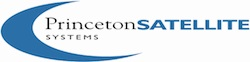# AerospaceUtils Module## Directory List

### AeroData

 AtmData.mat Atmospheric data binary file AtmData.txt Atmospheric data text file Cube.obj OBJ model of a cube with materials data. NRLMSIS.mat Data needed by AtmNRLMSISE SimpleSC.mat Simple MAT file for demos.

### AtmosphericCalculations

 AirData Computes air data based on a simplified standard atmosphere model. AtmDens1 Computes the atmospheric density using an exponential model. AtmDens2 Computes the Earth's atmospheric density using scale heights. AtmDensTitan Computes the atmospheric density of Titan using a curve fit. AtmGamma Computes the ratio of specific heats for air. AtmJ70 Computes the atmospheric density using Jacchia's 1970 model. AtmNRLMSISE NRLMSISE-00 Model 2001 for atmospheric density AtmSnd Computes the speed of sound in the atmosphere. AtmTemp Computes the atmospheric temperature using a simplified model. BaromExp Computes the barometric exponent. DiffusionExp Computes the diffusion exponent. JacchiaLineberry Jacchia-Lineberry model of the upper atmosphere. LocalSolarTime Compute local solar time NeptuneDensity Compute the atm. density (kg/m^3) on Neptune given the altitude (km) ReynoldsNumber Reynold's number as a function of atmospheric density. SutherlandsLaw Sutherland's law for dynamic viscosity. TemperatureStagnation Compute the stagnation temperature in the earth's atmosphere. UranusDensity Compute the atmospheric density on Uranus.

### Coord

 AzCoElToU Converts azimuth and coelevation to a unit vector. AzElRangeRangeDotToRV Compute r, v from range, range rate, az and el. AzElToU Converts azimuth and elevation to a unit vector. BankAngle Bank angle computations CoordinateTransform Transform between selected coordinate frames and representations. DownrangeAndAltFromTarget Finds the altitude and downrange from a target on a planet. ECEFToLLA Compute latitude, longitude, altitude from ECEF position. ECIToEF Computes the matrix from mean of Aries 2000 to the earth fixed frame. EFToLatLonAlt Convert an earth fixed position vector to [latitude;longitude;altitude] FLagrange Lagrange point RHS. Gc2GdLat Convert geocentric latitude to geodetic latitude. Gd2GcLat Convert geodetic latitude to geocentric latitude HorizonAngle Angle between the horizon and a vector from rG to rS. IntersectPlanet Altitude of the nearest point to a sphere. LLAToECEF Compute ECEF position from latitude, longitude, altitude. LatLonAltToEF Convert [latitude;longitude;altitude] to an earth fixed position vector. LatLonToR Converts geodetic latitude and longitude to r for an ellipsoidal planet. OmegaFromU Finds a total angular rate from a set of unit vectors. OmegaLVLH Generate the LVLH angular rate vector. Q2RADec Compute right ascension and declination of a vector from a quaternion. QAcq Given at least three vectors computes the initial quaternion. QAlign Rotate about a body axis to align a body vector with an inertial vector. QFrom2U Finds a quaternion with u1 aligned with u1ECI and u2 close to u2ECI. QHills Generate the quaternion that transforms from the ECI to the Hills frame. QIToBDot Computes the time derivative of a quaternion. QLVLH Generate the quaternions that transform from ECI to LVLH coordinates. QLatLon Generate the quaternions that transform from ECI to a lat/long frame QNadirPoint Generate a quaternion that points a body vector uB towards nadir. QRotateToAlign Rotate about an axis to align "ua" as close as possible to target "ut" QSLERP Interpolate a quaternion using the SLERP algorithm. QToPFTarget Generate a quaternion to go to a planet fixed lat/long target. QVectorAlign Rotate about an axis to align "ua" as close as possible to target "ut" R2LatLon Computes geocentric latitude and longitude from r RVToRADec Computes right ascension and declination and their rates. RaDec2Q Right ascension and declination to a quaternion. RollPitchToQECI Convert roll, pitch, r and v to QECIToBody SunAzEl Approx. azimuth and elevation of the sun for a time and place on earth. TargetFromRPVD Finds a target on a planet. U2AzEl Converts a unit vector to azimuth and elevation. U2RADec Computes the right ascension and declination from a unit vector.